IF YOU CAN SEE THIS go to /sfa/Nuprl/Shared/Xindentation_hack_doc.html

### Propositional Levels and Predicativity

As was mentioned in Higher-Order Propositions, even though one could characterize, say, disjunction or equality with a higher-order formula involving only implication and quantification over propositions, thus

Thm* A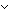B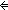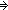(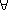C:Prop. (A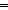C(BCC)
Thm*a,b:Aa = b(P:(A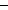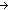Prop). P(aP(b))

we cannot adopt these as definitions, although it is not unusual to do so in some other accounts of higher-order logic. The reason is that we have adopted a "predicative" semantics (a term of Bertrand Russell's of uncertain etymology) which avoids defining a proposition by quantifying over a domain that includes the proposition to be defined. Basically, in a predicative logic, to assign meaning to a quantificational expression one must "already" have given meaning to all the expressions that might be used as witnesses. So, assigning a meaning to "C:Prop. (AC(BCC" requires already having assigned a meaning to every member of Prop, which therefore could not include "C:Prop. (AC(BCC" itself as a member. Thus, there is no type of all propositions in predicative type theory.

The solution adopted here is to stratify the propositions into a hierarchy Prop{i} where "i" is some positive number. Then

Thm*A,B:Prop{i}. (C:Prop{i}. (AC(BCC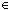Prop{i'}
Thm*A:Type{i}, a,b:A. (P:(AProp{i}). P(aP(b))Prop{i'}

where Prop{i'} is the next level of propositions above Prop{i}. The propositional levels are treated identically to the type levels explained in Universes and in Type Functionality Sequents. But disjunction and equality do not raise the propositional level, so the "iffs" of their higher-order characterizations cannot be converted to definitions:

Thm*A,B:Prop{i}. (ABProp{i}
Thm*T:Type{i}, a,b:T. (a = bTProp{i}

As with Universe Levels we normally elide the proposition level from display when it just a single level variable used throughout the expression. See Propositions for examples.

Quantification over types raises the propositional level, just as does quantification over propositions, indeed, "Type{[level]}" and "Prop{[level]}" are interchangeable as far as determining levels is concerned.

Thm* Prop{i}Type{i'}
Thm* Prop{i}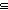Prop{i'}

Because the universes and propositional types are cumulative, one can often state theorems with a single level variable. For example,

Thm*A:Type{k}, P:(AProp{j}). (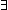x:AP(x))Prop{[k | j]}

which says that the existential is a proposition at the maximum of the two levels of its domain and predicate, follows simply from the at-first-sight less general

Thm*A:Type{i}, P:(AProp{i}). (x:AP(x))Prop{i}

since if AType{k} and PAProp{j} then AType{[k | j]} and PAProp{[k | j]}, putting (x:AP(x))Prop{[k | j]}. Of course, eliding the single level variable from the display of the last theorem gives us our usual

Thm*A:Type, P:(AProp). (x:AP(x))Prop